# WBJEE Maths Test - 2

## 75 Questions MCQ Test WBJEE Sample Papers, Section Wise & Full Mock Tests | WBJEE Maths Test - 2

Description
Attempt WBJEE Maths Test - 2 | 75 questions in 120 minutes | Mock test for JEE preparation | Free important questions MCQ to study WBJEE Sample Papers, Section Wise & Full Mock Tests for JEE Exam | Download free PDF with solutions
QUESTION: 1

Solution:
QUESTION: 2

Solution:
QUESTION: 3

### x=7 touches the circle x2 + y2 - 4x - 6y - 12 = 0, then the co-ordinates of the point of contact are

Solution:
QUESTION: 4

If in the expansion of ((x4) - (1/x3))15, x-17 occurs in the rth term, then

Solution:
QUESTION: 5
[(-1 + √(-3))/2]3n +[(-1 - √(-3))/2]3n is equal to
Solution:
QUESTION: 6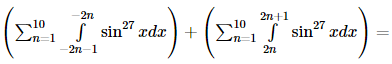Solution:
QUESTION: 7

x2 + y2 + 2(2K+3)x - 2Ky +(2K+3)2 + K2 - r2 = 0 represents the family of circles with centres on the line

Solution:
QUESTION: 8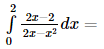Solution:
QUESTION: 9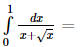Solution:
QUESTION: 10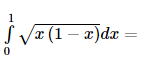Solution:
QUESTION: 11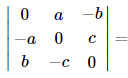Solution:
QUESTION: 12

The solution of the equation (1+x2)(1+y)dy+(1+x)(1+y2)dx=0 is

Solution:
QUESTION: 13

The differential equation of family of curves y=a cos(x+b) is

Solution:
QUESTION: 14

If z 1 and z 2 are two non-zero complex numbers such that |z1 + z2| = |z1| + |z2| , then Arg z1 − Arg z2 is

Solution: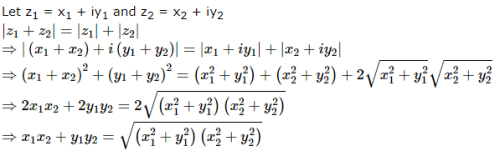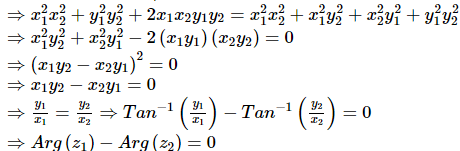QUESTION: 15

The differential of sin⁻1[(1-x)/(1+x)] w.r.t. √x is equal to

Solution:

Let u = sin-1(1 – x)/(1 + x)

v = √x

Differentiate w.r.t.x

du/dx = [1/√(1 – (1 – x)2/(1 + x)2 ][d/dx (1 – x)/(1 + x)]

= (1+ x)/√((1 + x)2 – (1 – x)2) [ (1 + x) ×-1 – (1-x)]/(1 + x)2

= (1/√4x)×[ -1 – x – 1 + x]/(1 + x)

= -2/√(4x)(1 + x)

= -1/√x(1 + x)

dv/dx = 1/2√x

du/dv = (du/dx)/(dv/dx)

= -2/(1 + x)

QUESTION: 16

The function f(x)=|x| is defined on [-1,1]. It does not satisfy the Rolle's theorem because

Solution:
QUESTION: 17

In an ellipse the distance between the foci is 6 and it's minor axis is 8. Then its eccentricity is

Solution:
QUESTION: 18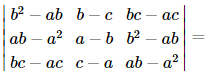Solution: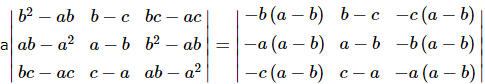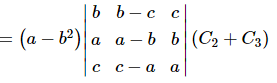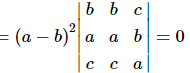QUESTION: 19

If S′ and S are the foci of the ellipse (x2/a2)+(y2/b2)=1 and P(x,y) be a point on it, then the value of SP + S′P is

Solution:
QUESTION: 20
The vertices of a hyperbola are (2, 0), (-2, 0) and the foci are (3, 0), (-3, 0). The equation of the hyperbola is
Solution:
QUESTION: 21

If e and e ′ are the eccentricities of the hyperbola x2 ∕ a2 − y2 ∕ b2 = 1 and its conjugate hyperbola, the value of 1 ∕ e2 + 1 ∕ e′2 is

Solution:
QUESTION: 22

Sin (sin⁻11/2 + cos⁻11/2) equals

Solution:
QUESTION: 23

The equation of circle which passes through (4,5) and whose centre is (2,2) is

Solution:
QUESTION: 24

If the function f (x)   =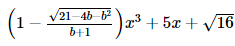increases for all x, then

Solution:

Since the function f(x) increases for all x, therefore,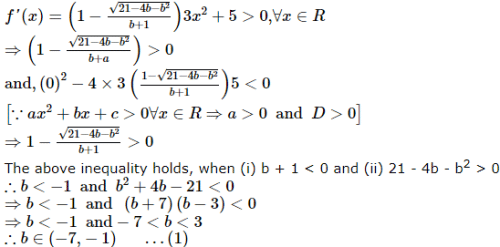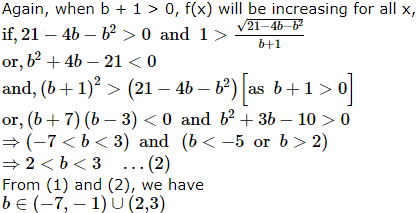QUESTION: 25
If 2 cos θ =x+1/x and 2 cos φ =y+1/y, then the value of cos (θ+φ) will be
Solution:
QUESTION: 26

If the function f(x) = 2x3 - 9ax2 + 12a2x + 1, where a > 0, attains its max. and min. at p and q respectively such that p2 = q then a equals

Solution:
QUESTION: 27

If A is a square matrix such that A2 = I, then A⁻1 is equal to

Solution:
QUESTION: 28

If the parabola y2=4ax passes thro' the point (1,-2), then the tangent at this point is

Solution:

Since the parabola y2=4ax passes through the point (1,−2),
∴(−2)2=4a(I)⇒a=1
Equation of tangent to the parabola at (1,−2)
yy1​=2a(x+x1​) or
y(−2)=2(1)(x+1) or x+y+1=0

QUESTION: 29

The line x-y+2=0 touches the parabola y2=8x at the point

Solution:
QUESTION: 30

The number of 7 digit numbers which can be formed using the digits 1, 2, 3, 2, 3, 3, 4 is

Solution:

There are 7 digits 1, 2, 3, 2, 3, 3, 4 in which 2 occurs 2 times and 3 occurs 3 times
Number of 7 digit numbers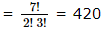QUESTION: 31

How many words are formed from the letters of the word EAMCET so that two vowels are never together?

Solution:

Reqd ways =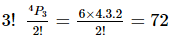QUESTION: 32
There are ten bulbs in a hall. Each one of them can be switched on independently. The number of ways of illuminating the hall is
Solution: Every bulb can be operated in two ways
There is only one condition when hall is not illuminaled that is no bulb is switched on
∴ No. of ways of illuminating hall
= 210 - 1 = 1023
QUESTION: 33

The probability of getting a total of 10 in a single throw of two dice is

Solution:
QUESTION: 34

A fair dice is tossed repeatedly until six shows up 3 times. The probability that exactly 5 tosses are needed is

Solution:
QUESTION: 35

The probability that a radar will detect an object is p. The probability that it will be detected in n cycles is

Solution:
QUESTION: 36

Solution:

The exradius =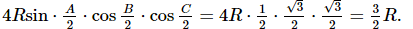QUESTION: 37

If the side of a triangle are 13, 14, 15, then the radius of the incircle is

Solution:
QUESTION: 38

Let α, β be the roots of the quadratic equation x2 + px + p3 = 0. If (α, β) is a pt. on the parabola y2 = x, then the roots of the qudratic equation are

Solution:
QUESTION: 39
If a, b, c, d, e, f are in A.P. then e - c is equal to
Solution:
QUESTION: 40

The absiccae of the points of the curve y = x3 in the interval [-2, 2], when the slope of the tangent can be obtained by mean value theorem for the interval [-2, 2] are

Solution:

Given that equation of curve y = x3 = f (x)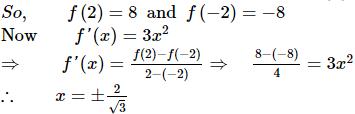QUESTION: 41

If a, b, c are distinct +ve real numbers and a2 + b2 + c2 = 1 then ab + bc + ca is

Solution:
QUESTION: 42
In the expansion of (1 + x)n,coefficients of 2nd ,3rd and 4th terms are in A.P.Then n is equal to
Solution:
QUESTION: 43

If the roots of the equation x3 - 12x2 + 39x - 28 = 0 are in A.P., then their common difference will be

Solution:
QUESTION: 44
The sum of integers from 1 to 100 which are divisible by 2 or 5 is
Solution:
QUESTION: 45

The function f (x) = log ((1 + x)/(1 - x)) satisfies the equation

Solution:
QUESTION: 46

If A = [(x,y) : x2 + y2 = 25] and B = [(x,y) : x2 + 9y2 = 144] and A ∩ B contains

Solution:
QUESTION: 47

The equation of bisectors between the lines 3x+4y-7=0 and 12x+5y+17=0 are

Solution:
QUESTION: 48
The equation of a line passing through (3,-4) and perpendicular to line 3x+4y=5 is
Solution:
QUESTION: 49

The roots of the equation x4 - 4x3 + 9x2 − 12x + 18 = 0 are____

Solution:
QUESTION: 50
If sin2θ=cos3θ and θ is an acute angle, sinθ=
Solution:
QUESTION: 51

The sides AB, BC and CA of a triangle ABC have 3, 4 and 5 interior points respectively on them. The number of triangles that can be constructed using these interior points as vertices is

Solution:
QUESTION: 52

If the curves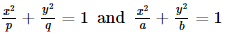intersect each other at right angles, then

Solution:
QUESTION: 53

Let the algebraic sum of the perpendicular distances from (3, 0), (0, 3) and (2, 2) to a variable line is zero, then the line passes through a fixed point whose co-ordinates are

Solution:
QUESTION: 54

Let d1 , d2 , d3 , … …, dk be all the divisors of a positive integer n including 1 and n.
Suppose d1 , d2 , d3 + … … + dk = 72 , then the
value of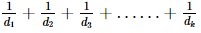is

Solution:
QUESTION: 55

The equation f(x) = 0 has eight distinct real solution f also satisfy f(4+x) = f(4-x). The sum of all the eight solution of f(x) = 0 is

Solution:
QUESTION: 56

In a triangle X Y Z , ∠ Z =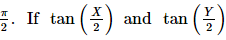are the roots of the equation ax2 + bx + c = 0, a ≠ 0 then

Solution:
QUESTION: 57

If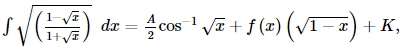, then

Solution:
QUESTION: 58

In triangle ABC, line joining circumcentre and incentre is parallel to side AC, then cos A + cos C is equal to

Solution:
QUESTION: 59

The system of confocal conics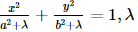being an arbitary constant

Solution:
QUESTION: 60

Two mutually perpendicular tangents of the parabola y2 = 4ax meet the axis in P1 and P2. If S is the focus then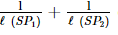equals

Solution:
QUESTION: 61

If a line segment AM = 'a' moves in the plane XOY remaining parallel to OX so that the left end point A slides along the circle x2 + y2 = a2, the locus of M is

Solution:
QUESTION: 62

Let P (a secθ , b tan θ)    and Q (a sec φ , b tan φ)  where θ + φ = π/2 , be two points on the hyperbola x2 ∕ a2 − y2 ∕ b2 = 1 . If (h, k) is the point of intersection of normals at P and Q, then k is equal to

Solution:
QUESTION: 63

Let g x =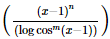;0 < x < 2, m and n are integers, m ≠0, n > 0 and let p be the left hand derivative of | x - 1 | at x = 1.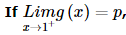then

Solution:
QUESTION: 64

The locus of the orthocentre of the triangle formed by the lines
(1 + p)x - py + p(1 + p) = 0,
(1 + q)x - qy + q(1 + q) = 0,
and y = 0, where p ≠ q , is

Solution:
QUESTION: 65

Let x be a positive real number not equal to 1. In each of the following diagrams, the shaded region does not include its boundary. Which of these regions represents the set {x,y| logx logxy2 > 0} ?

Solution:
*Multiple options can be correct
QUESTION: 66

The set of discontinuities of the function f (x) = ( 1/2 − cos2x) contains the set

Solution:
*Multiple options can be correct
QUESTION: 67

The value of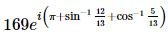is

Solution:
QUESTION: 68

In a triangle ABC, tan C < 0. Then

Solution:
*Multiple options can be correct
QUESTION: 69

Two functions f and g have first and second derivatives at x = 0 and satisfy the relations,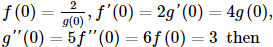Solution:
*Multiple options can be correct
QUESTION: 70

The differential equation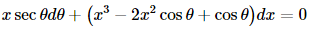Solution:
*Multiple options can be correct
QUESTION: 71

Let x0 = 2007 and let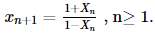Then

Solution:
*Multiple options can be correct
QUESTION: 72

If z1 = a + ib and z2 = c + id are complex number such that |z1| = |z2| = 1 and Re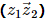= 0, then the pair of complex numbers w1 = a + ic and w2 = b + id satisfies :

Solution:
*Multiple options can be correct
QUESTION: 73

Let A and B be two square matrices of same order that satisfy A + B = 2B and 3A + 2B = l then which of the following statements is true?

Solution:
*Multiple options can be correct
QUESTION: 74

There exist a triangle ABC satisfying the conditions,

Solution:
*Multiple options can be correct
QUESTION: 75

If P is any point lying on the ellipse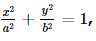whose foci are S and S'. Let ∠ PSS ′ = α and ∠ PS′S = β , then:

Solution:Use Code STAYHOME200 and get INR 200 additional OFF Use Coupon Code